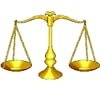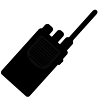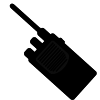Search by Topic

Resources tagged with Number bases similar to Binary Squares:

Filter by: Content type:
Age range:
Challenge level:

There are 17 results

Broad Topics > Numbers and the Number System > Number basesBinary Squares

Age 16 to 18 Challenge Level:

If a number N is expressed in binary by using only 'ones,' what can you say about its square (in binary)?Nim

Age 14 to 16 Challenge Level:

Start with any number of counters in any number of piles. 2 players take it in turns to remove any number of counters from a single pile. The loser is the player who takes the last counter.Balance Power

Age 11 to 18 Challenge Level:

Using balancing scales what is the least number of weights needed to weigh all integer masses from 1 to 1000? Placing some of the weights in the same pan as the object how many are needed?Nim-like Games

Age 7 to 16 Challenge Level:

A collection of games on the NIM themeA Story about Absolutely Nothing

Age 7 to 18

This article for the young and old talks about the origins of our number system and the important role zero has to play in it.Latin Numbers

Age 14 to 16 Challenge Level:

Can you create a Latin Square from multiples of a six digit number?Basic Rhythms

Age 16 to 18 Challenge Level:

Explore a number pattern which has the same symmetries in different bases.Phew I'm Factored

Age 14 to 16 Challenge Level:

Explore the factors of the numbers which are written as 10101 in different number bases. Prove that the numbers 10201, 11011 and 10101 are composite in any base.Composite Notions

Age 14 to 16 Challenge Level:

A composite number is one that is neither prime nor 1. Show that 10201 is composite in any base.Back to Basics

Age 14 to 16 Challenge Level:

Find b where 3723(base 10) = 123(base b).Learn about Number Bases

Age 11 to 18

We are used to writing numbers in base ten, using 0, 1, 2, 3, 4, 5, 6, 7, 8, and 9. Eg. 75 means 7 tens and five units. This article explains how numbers can be written in any number base.Knapsack

Age 14 to 16 Challenge Level:

You have worked out a secret code with a friend. Every letter in the alphabet can be represented by a binary value.Code to Zero

Age 16 to 18 Challenge Level:

Find all 3 digit numbers such that by adding the first digit, the square of the second and the cube of the third you get the original number, for example 1 + 3^2 + 5^3 = 135.Guesswork

Age 14 to 16 Challenge Level:

Ask a friend to choose a number between 1 and 63. By identifying which of the six cards contains the number they are thinking of it is easy to tell them what the number is.The Knapsack Problem and Public Key Cryptography

Age 16 to 18

An example of a simple Public Key code, called the Knapsack Code is described in this article, alongside some information on its origins. A knowledge of modular arithmetic is useful.Secret Transmissions

Age 14 to 16 Challenge Level:

How can Agent X transmit data on a faulty line and be sure that her message will get through?More Secret Transmissions

Age 16 to 18 Challenge Level:

In 'Secret Transmissions', Agent X could send four-digit codes error free. Can you devise an error-correcting system for codes with more than four digits?